### Calculating the time needed to run a straight between two turns in the micromouse contest

When a micromouse robot is trying to determine the best path from start to goal, it is helpful to be able to calculate the amount of time it will take to run along the straight path that connects two turns. This is not an obviously simple calculation because there is the question of how fast the robot is travelling at the start and end of the straight, the available acceleration and the maximum speed limit.

Some simplifying assumptions will help a lot. First, assume that the start and end speed for the straight is the same That is, all turns are made at the same speed. Second, assume that the robot is always either accelerating or decelerating. That is, there is no top speed limit. For many actual straight section in the maze, that is likely to be the case. Finally, assume that the acceleration and deceleration is the same so that the straight run is symmetrical.

## Orthogonal moves

The classical equations of motion give an expression for the time taken to move by a distance,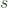, if the acceleration,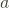, and start speed,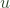, are known (suvat-rearranged)

(1)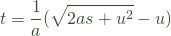Considering orthogonal moves first, the distance,, can be expressed as some multiple,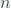, of the length of one cell,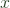.

(2)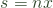Substitute into (1) to get:

(3)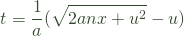It is now convenient to specify the start speed,, as some constant,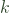, times the distance through one cell.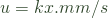and the acceleration,, as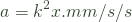For example, for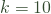, a classic micromouse might be able to turn at 1800mm/s and accelerate at 18,000 mm/s/s.

Substituting into (2):

(4)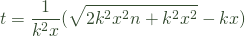)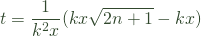(6)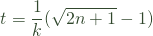Equation 6 describes the time taken to accelerate to some top speed overcells. The micromouse robot will accelerate for half that number of cells and then brake for the remaining half. Split the motion into two parts: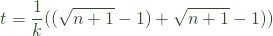(7)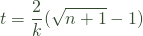This result is easily pre-calculated in a table of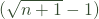for suitable values ofand so it will be easy to determine the time needed to run any straight or arbitrary length with a simple multiply. Note that this only works because of the common factor,, in the speed and acceleration.

## Diagonals

For diagonal movements, each cell traversed is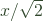mm long and so the time is calculated from:

(8)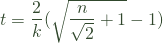Note that you cannot simply multiply the result from equation (7) by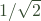.

This site uses Akismet to reduce spam. Learn how your comment data is processed.